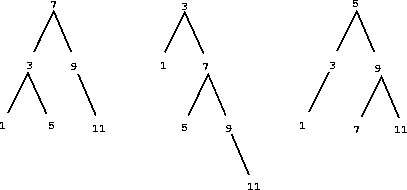# Converting a binary tree to a list

Each of the following two procedures converts a binary tree to a list.

``````(define (tree->list-1 tree)
(if (null? tree)
'()
(append (tree->list-1 (left-branch tree))
(cons (entry tree)
(tree->list-1 (right-branch tree))))))

(define (tree->list-2 tree)
(define (copy-to-list tree result-list)
(if (null? tree)
result-list
(copy-to-list (left-branch tree)
(cons (entry tree)
(copy-to-list (right-branch tree)
result-list)))))
(copy-to-list tree '()))
``````

a. Do the two procedures produce the same result for every tree? If not, how do the results differ? What lists do the two procedures produce for the trees in figure 2.16?

b. Do the two procedures have the same order of growth in the number of steps required to convert a balanced tree with `n` elements to a list? If not, which one grows more slowly?Figure 2.16: Various binary trees that represent the set `{1, 3, 5, 7, 9, 11}` .